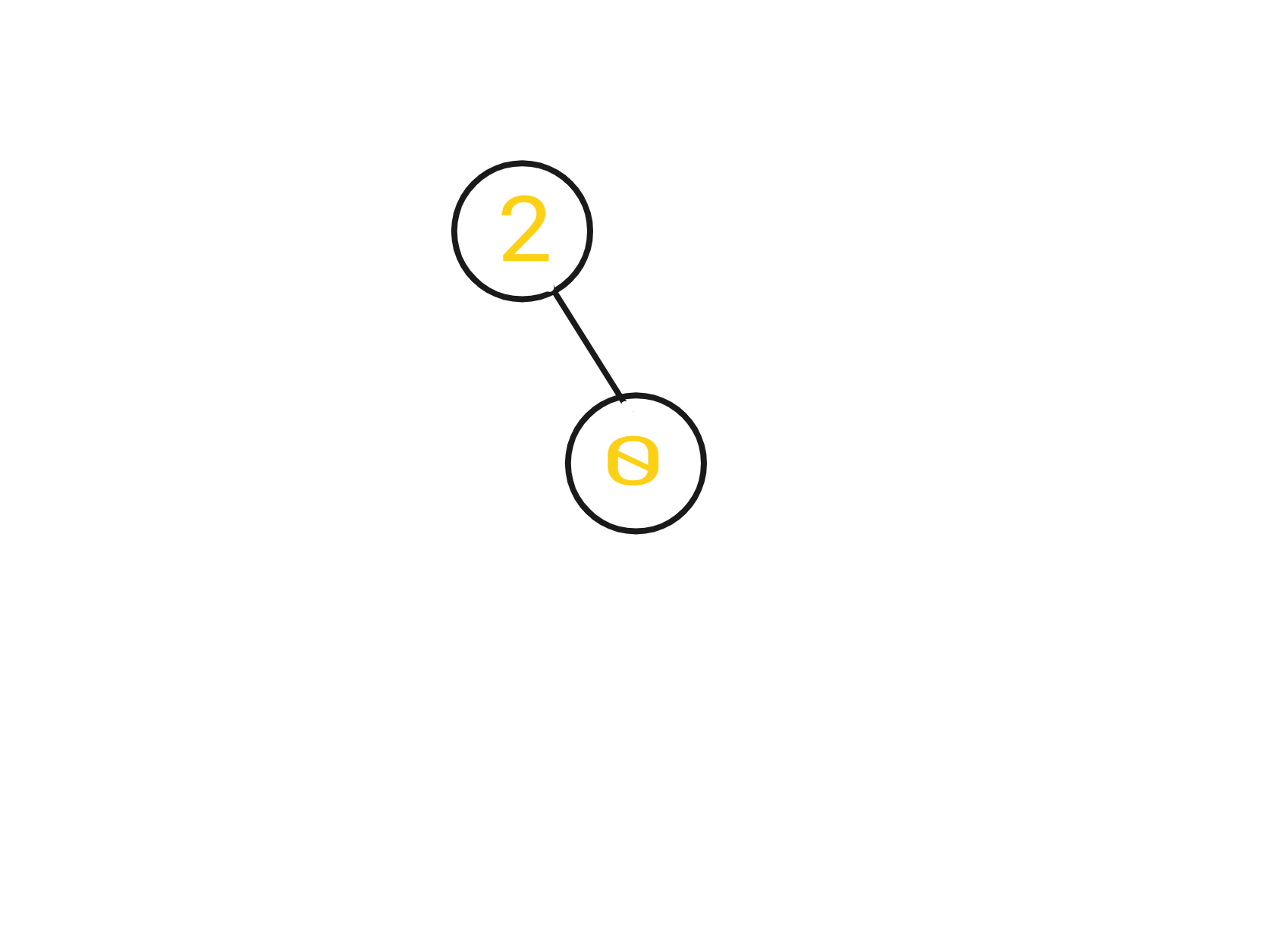New update is available. Click here to update.
Last Updated: 22 Mar, 2021
##### Distributing Coins
Moderate
Problem statement#### Note:

``````A move may be from parent to child or from child to parent.
``````

#### For example,

``````Given ‘ROOT’ = [2,-1,0,-1,-1]

The tree would look like this :
````````````The answer would be 1, because the root node will transfer 1 coin to its right child. Thus both nodes have the same number of coins now.
``````

#### Input format :

``````The first line of input contains an integer ‘T’ denoting the number of test cases.

In the next T lines, the first line contains each test case contains space-separated integers denoting the nodes of the first binary tree, where -1 indicates that the NULL pointer has been appointed to the previous node.

The input is given in a preorder way, that is, the node then left subtree, and then right subtree as shown in the example.
``````

#### Output format :

``````For each test case, print a single line containing a single integer denoting the number of coins moved.

The output of each test case will be printed in a separate line.
``````

#### Note:

``````You do not need to print anything. It has already been taken care of. Just implement the given function.
``````

#### Constraints:

``````1 <= T  <= 100
1 <=  N <= 10^5
-10^6 <= DATA <= 10^6

Where ‘T’ is the number of test cases and  ‘N’ denotes the number of nodes in the binary tree, and ‘DATA’ represents the value of the node.

Time limit: 1 sec.
``````Approaches

## 01ApproachThe main idea is to use recursion to traverse the tree in a pre-order way and do the following :

• Go to the left subtree and figure how much do we need or if we have got extra? I'll give that/take it away to/from you via our direct edge and pass it to the right child, and if something remains, I'll take it.
• Go to the right subtree and figure how much do we need or if we have got extra? I'll give that/take it away to/from you via our direct edge and pass it to the right child, and if something remains, I'll take it.
• The answer will be the sum of values(absolute) returned after the asked questions from the left subtree and right subtree.
• But what should the root return to its parent? It will return how much "its subtree" needs/has extra. That will be the signed sum of its Left+Right (question's answer) + same question asked to the current root node.